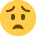Current updates regarding coronavirus (Covid-19) and the precautions AAT are taking will be continually updated on the below page.

We hope you are all safe and well and if you need us we will be here. 💚

# Elements of Costing help!

Hello

One of the questions on the practice exam is the below, and i am stuck on how to answer it. The books I'm using sets it out in a totally different way and it suddenly makes no sense to meHardCast Ltd is looking to calculate the unit cost for one of the products it makes. It needs to calculate an overhead absorption rate to apply to each unit.
per machine hour
per labour hour
per unit

Total factory activity for all production is forecast as follows:

Machine hours = 20,000
Labour hours = 25,000
units = 120,000

(a) complete the table to show the possible overhead absorption rates.

Machine hour Labour hour unit

activity
absorption rate £

The following data relates to making one unit of the product

material - 4kgs at £5 ker kilo
labour - 15 mins at £20 per hour
production time - 12 mins

(b) complete the table below to calculate the total unit cost, using the three overhead absorption rates you have calculated in a

cost machine hour £ labour hour£ unit£

material
labour
direct cost
total unit cost

• below is a screenshot of the actual questions, might be easier to read then what i typed!!!

Machine hr (where overhead is £300,000 and activity is 20,000 machine hrs)
Absorption rate = £300,000/20,000 machine hrs = £15.00 per machine hrs

Labour hr (where overhead is £300,000 and activity is 25,000 labour hrs)
Absorption rate = £300,000/25,000 labour hours = £12.00 per labour hr

Unit (where overhead is £300,000 and activity is 120,000 units)
Absorption rate = £300,000/120,000 units = 2.50 per unit

b) Calculate the standard cost (cost for one unit) from the information given.

Material (4 kg at £5 per kg) one unit uses 4kg and at £5 per kg cost a total of £20.00 per unit

Labour (15 mins at £20 phr) one unit uses 15/60 = 0.25hr and at £20 phr cost a total £5.00 per unit

Direct cost per unit is these two added together for the three options.

Unit method
This is straight forward as the answer is already per unit £2.50 per unit
Total unit cost = £25.00 + £2.50 = £27.50

Labour hr method
Each unit takes 15 mins of labour. Where absorption rate is £12 per labour hr, one unit costs 15/60 x £12 = £3.00
Total unit cost = £25.00 + £3.00 = £28.00

Machine hr method
Each unit takes 12 mins of production time. Where absorption rate is £15 per machine hr, one unit costs 12/60 x £15 = £3.00
Total unit cost = £25.00 + £3.00 = £28.00

Important: show answers to two decimal places. This is easy to overlook and marks will be lost if not followed.
• Amazing! Thank you so much, this question was giving me so much greif!!!